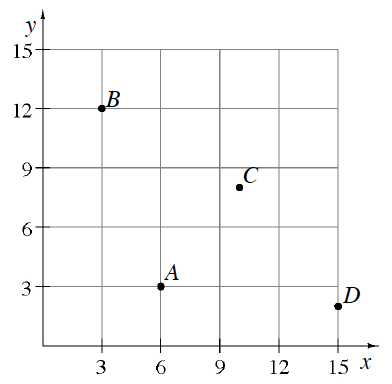### Home > ACC6 > Chapter 6 Unit 6 > Lesson CC1: 6.1.4 > Problem6-51

6-51.

Name the coordinates of each point shown in the graph at right using ordered pairs $(x, y)$.

$A=(6,3)$ and $B=(3,12)$.

Complete the ordered pairs for $C$ and $D$.